If you find any of this useful, please consider donating via PayPal to help keep this site going.# Using Pseudo-random Numbers to Solve Mathematical Problems

6/5/11

For this article, I'll discuss a technique called Monte Carlo Integration (MCI) that relies on pseudo-random numbers.

Around 1946, von Neumann, Fermi, and Ulam had the idea for the Monte Carlo method. Ulam recounts:

"The first thoughts and attempts I made to practice [the Monte Carlo Method] were suggested by a question which occurred to me in 1946 as I was convalescing from an illness and playing solitaires. The question was what are the chances that a Canfield solitaire laid out with 52 cards will come out successfully? After spending a lot of time trying to estimate them by pure combinatorial calculations, I wondered whether a more practical method than "abstract thinking" might not be to lay it out say one hundred times and simply observe and count the number of successful plays. This was already possible to envisage with the beginning of the new era of fast computers, and I immediately thought of problems of neutron diffusion and other questions of mathematical physics, and more generally how to change processes described by certain differential equations into an equivalent form interpretable as a succession of random operations. Later [in 1946, I] described the idea to John von Neumann, and we began to plan actual calculations".
(from Eckhardt, Roger (1987). Stan Ulam, John von Neumann, and the Monte Carlo method, Los Alamos Science, Special Issue (15), 131-137)

Consider a basic problem of calculus, that of finding the area, A, underneath a curve on an interval [a,b]. This is represented by the definite integral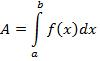This integral can be approximated by a sum, such as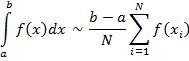This approximation is quite useful for complicated f(x), and it is called a Riemann sum. It amounts to partitioning the interval [a,b] into subintervals, choosing which xi to evaluate in the subintervals, and approximating the area underneath the curve (which can be difficult) by the sum of the areas of more and more rectangles which get thinner and thinner. The latter is easy to do because Area = Base*Height for any rectangle.

Consider approaching this problem in a very different way; using probability.

As an illustration of MCI, let's estimate the area of a circle with radius 1. Recall that the formula for the area of a circle with radius r is A = pi*r2, so with r = 1, A = pi. The equation of a circle of radius r, centered at the origin, is r2 = x2 + y2. For a unit circle, r = 1, so we get 1 = x2 + y2. Note that f(x)=(1-x2).5 is the quarter of a unit circle in the upper-right quadrant. Therefore, the area under this curve in the upper-right quadrant, multiplied by 4, would be an estimate of pi.

Let's "throw darts" at this graph and count the proportion of darts that land inside the curve. Because the curve is embedded in a 1 x 1 box, and the total area of that box is 1, the proportion of darts that land inside the curve will estimate the area under the curve which is the area of a quarter of a circle. Multiplying this estimate by 4 would then give us our estimate of pi. This "throwing darts" approach is called "crude MCI". Notice with MCI that the xi's, and therefore the f(xi)'s, are random.

Let's start by throwing N = 1 point. Here we go!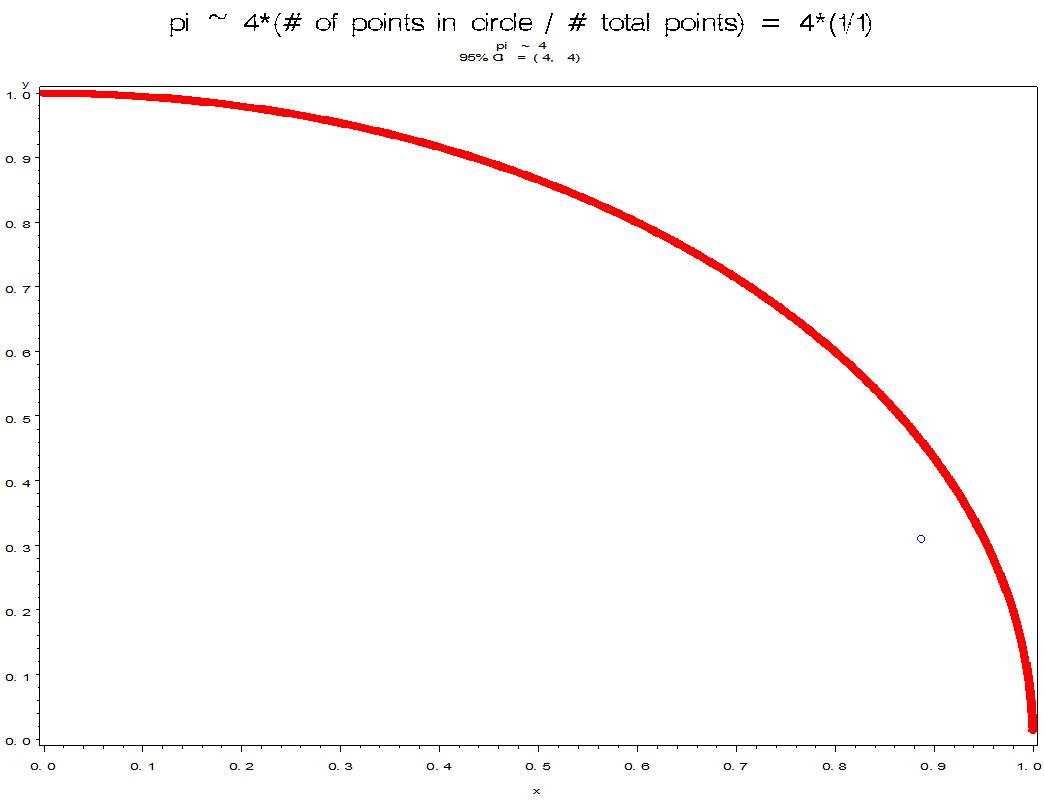Note that 4 is not a very good estimate of pi. This is not surprising, because N is only 1. Let's throw some more points.This is starting to look promising with N = 100 and an estimate of 2.96 and a 95% confidence interval of [2.61, 3.31].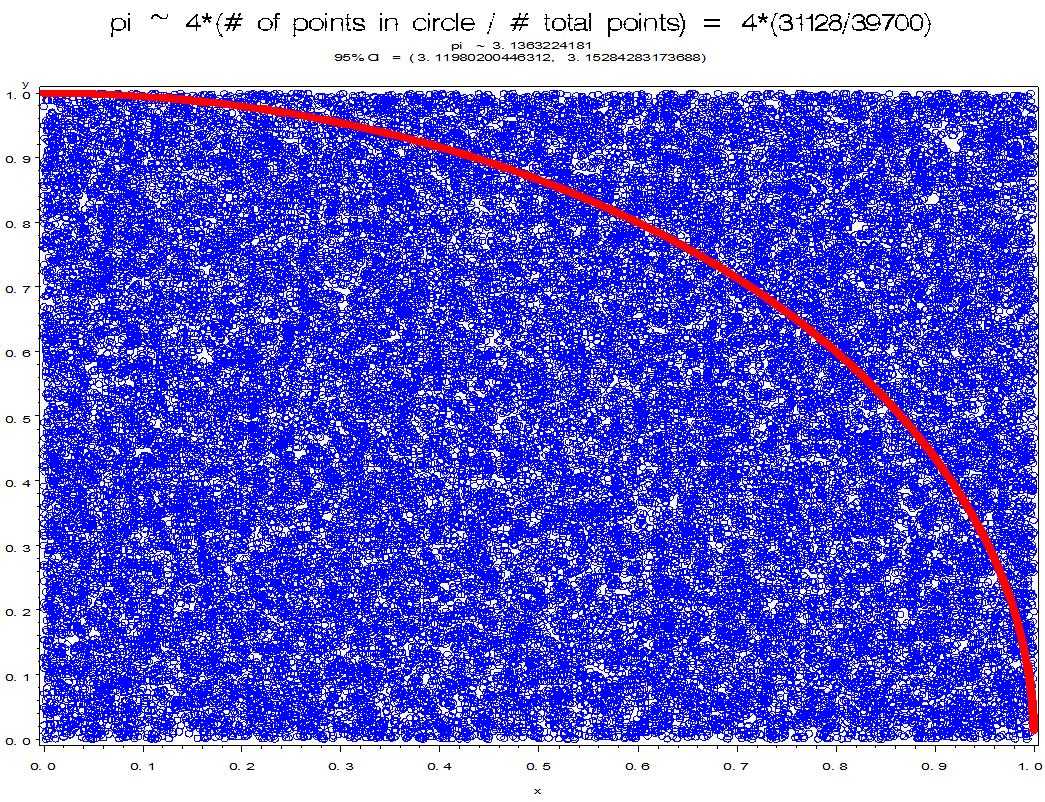The picture looks much better with N = 39,700 and the estimate of 3.136 and a 95% confidence interval of [3.12, 3.15]. Notice how the points are filling in the area.

Now that you have the idea, let's use N = 1 to N = 50,000 and plot the resulting estimates of pi and a confidence interval around each estimate.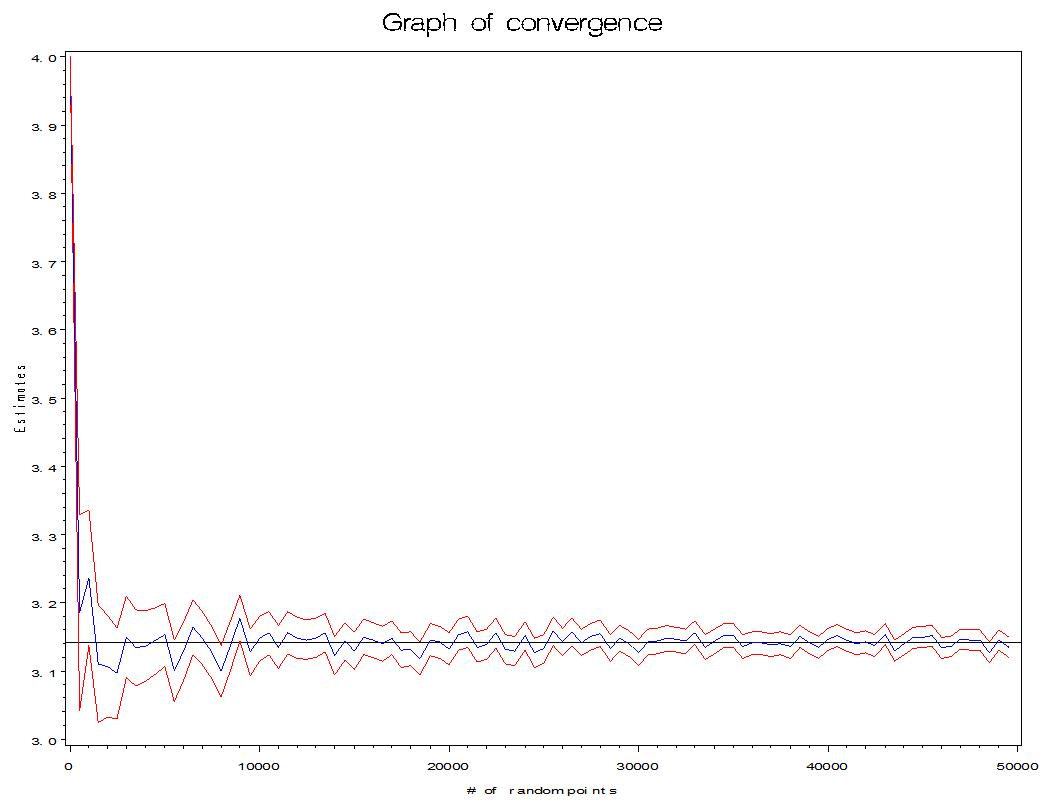Note that the black horizontal line is at 3.14159.

It appears that the estimates are getting closer to pi and the confidence intervals are getting narrower as N increases. In fact, the Law of Large Numbers guarantees that the MCI estimate will converge to pi as N gets larger and larger. However, because of a slow rate of convergence, improvements have been made on "crude MCI". This blog is too short to go into all the details of these improvements, but the reader can look up "Importance Sampling", "Control Variates", and "Stratified Sampling". Just note that crude MCI and the improvements arise from sampling theory and variance reduction techniques.

Who would have thought that sampling theory could be used to solve problems in areas other than Censuses and Surveys?